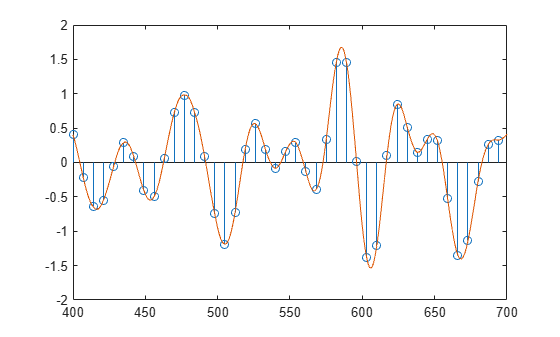# intfilt

Interpolation FIR filter design

## Syntax

``b = intfilt(l,p,alpha)``
``b = intfilt(l,n,'Lagrange')``

## Description

example

````b = intfilt(l,p,alpha)` designs a linear phase FIR filter that performs ideal bandlimited interpolation using the nearest `2`*`p` nonzero samples, when used on a sequence interleaved with `l`-1 consecutive zeros every `l` samples, assuming an original bandlimitedness of `alpha` times the Nyquist frequency. The returned filter `b` is identical to that used by `interp`.```
````b = intfilt(l,n,'Lagrange')` designs an FIR filter that performs `n`th-order Lagrange polynomial interpolation on a sequence interleaved with `l`-1 consecutive zeros every `l` samples.```

## Examples

collapse all

Design a digital interpolation filter to upsample a signal by seven, using the bandlimited method. Specify a "bandlimitedness" factor of 0.5 and use $2×2$ samples in the interpolation.

```upfac = 7; alpha = 0.5; h1 = intfilt(upfac,2,alpha);```

The filter works best when the original signal is bandlimited to `alpha` times the Nyquist frequency. Create a bandlimited noise signal by generating 200 Gaussian random numbers and filtering the sequence with a 40th-order FIR lowpass filter. Reset the random number generator for reproducible results.

```lowp = fir1(40,alpha); rng('default') x = filter(lowp,1,randn(200,1));```

Increase the sample rate of the signal by inserting zeros between each pair of samples of `x`.

`xr = upsample(x,upfac);`

Use the `filter` function to produce an interpolated signal.

`y = filter(h1,1,xr);`

Compensate for the delay introduced by the filter. Plot the original and interpolated signals.

```delay = mean(grpdelay(h1)); y(1:delay) = []; stem(1:upfac:upfac*length(x),x) hold on plot(y) xlim([400 700])````intfilt` also performs Lagrange polynomial interpolation.

• First-order polynomial interpolation is just linear interpolation, which is accomplished with a triangular filter.

• Zeroth-order interpolation is accomplished with a moving average filter and resembles the output of a sample-and-hold display.

Interpolate the original signal and overlay the result.

```h2 = intfilt(upfac,1,'Lagrange'); y2 = filter(h2,1,xr); y2(1:floor(mean(grpdelay(h2)))) = []; plot(y2) hold off```## Input Arguments

collapse all

Number of samples, specified as a positive integer scalar. `intfilt` designs a linear phase FIR filter using a sequence interspersed with `l`-1 consecutive zeros every `l` samples.

Number of nonzero samples, specified as a positive integer scalar. `intfilt` designs a linear phase FIR filter that performs bandlimited interpolation using the nearest 2*`p` nonzero samples.

Inverse measure of transition bandwidth, specified as a scalar. `alpha` is inversely proportional to the transition bandwidth of the filter and it also affects the bandwidth of the don't-care regions in the stopband. Specifying `alpha` allows you to specify how much of the Nyquist interval your input signal occupies. This is beneficial for signals to be interpolated because it allows you to increase the transition bandwidth without affecting the interpolation and results in better stopband attenuation for a given `l` and `p`. If you set `alpha` to 1, your signal is assumed to occupy the entire Nyquist interval. Setting `alpha` to less than one allows for don't-care regions in the stopband. For example, if your input occupies half the Nyquist interval, you could set alpha to 0.5.

Order of Lagrange polynomial specified as a positive integer scalar. The FIR filter performs `n`th-order Lagrange polynomial interpolation on a sequence interleaved with `l`-1 consecutive zeros every `l` samples. If both `n` and `l` are even, the filter designed is not linear phase.

Polynomial interpolation method, specified as 'Lagrange'.

## Output Arguments

collapse all

Filter coefficients, returned as a vector. Elements of `b` are the coefficients of an FIR filter. If `alpha` is specified, it assumes an original bandlimitedness of `alpha` times the Nyquist frequency. `b` is length 2*`l`*`p`-1.

For the `n`th-order Lagrange polynomial interpolation, `b` has length (`n`+1)*`l` for `n` even, and length (`n`+1)*`l-1` for `n` odd.

## Algorithms

The bandlimited method uses `firls` to design an interpolation FIR filter. The polynomial method uses Lagrange's polynomial interpolation formula on equally spaced samples to construct the appropriate filter. Both types of filters are basically lowpass and have a gain of `l` in the passband.